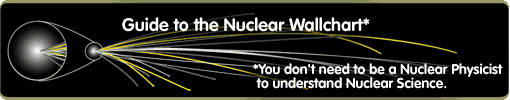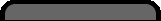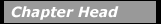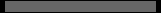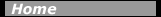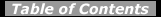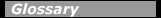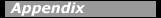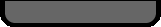## Four Fundamental InteractionsThe forces of gravity and electromagnetism are familiar in everyday life. Two new forces are introduced when discussing nuclear phenomena: the strong and weak interactions. When two protons encounter each other, they experience all four of the fundamental forces of nature simultaneously. The weak force governs beta decay and neutrino interactions with nuclei. The strong force, which we generally call the nuclear force, is actually the force that binds quarks together to form baryons (3 quarks) and mesons (a quark and an anti-quark). The nucleons of everyday matter, neutrons and protons, consist of the quark combinations uud and udd, respectively. The symbol u represents a single up quark, while the symbol d represents a single down quark.

The force that holds nucleons together to form an atomic nucleus can be thought to be a residual interaction between quarks inside each individual nucleon. This is analogous to what happens in a molecule. The electrons in an atom are bound to its nucleus by electromagnetism: when two atoms are relatively near, there is a residual interaction between the electron clouds that can form a covalent bond. The nucleus can thus be thought of as a "strong force molecule."

The force between two objects can be described as the exchange of a particle. The exchange particle transfers momentum and energy between the two objects, and is said to mediate the interaction. A simple analogue of this is a ball being thrown back and forth between two people. The momentum imparted to the ball by one person gets transferred to the other person when she catches the ball.

Table 4-1. Strength and range of the four fundamental forces between two protons. Note that the strong force acts between quarks by an exchange of gluons. The residual strong force between two protons can be described by the exchange of a neutral pion. Note, the W± is not included as an exchange particle for the weak interaction because it is not exchanged in the simplest proton-proton interaction.

 Interaction Gravity Weak Electro-magnetism Strong Residual Exchange particle Graviton Z0 Photon Pion Mass mc2 (eV) 0 91x109 0 135x106 Coupling constant C2 (J · m) 1.87x10-64 3.22x10-31 2.31x10-28 2.5x10-27 Range (m) infinity 2x10-18 infinity 1.5x10-15

The potential energy associated with each force acting between two protons is characterized by both the strength of the interaction and the range over which the interaction takes place. In each case the strength is determined by a coupling constant, and the range is characterized by the mass of the exchanged particle. The potential energy, U, between two protons a distance r apart is written as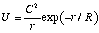,

where R is the range of the interaction, and C2, is the strength of the interaction. In each case the interaction is due to the exchange of some particle whose mass determined the range of the interaction, R = h/mc. The exchanged particle is said to mediate the interaction.

Table 4-1 shows a comparison between the coupling constants and ranges of the four forces acting between two protons. Although the graviton has not yet been observed, it is thought that there is an exchange particle associated with gravity and that eventually gravity will be described in a unified theory with the other three forces of nature.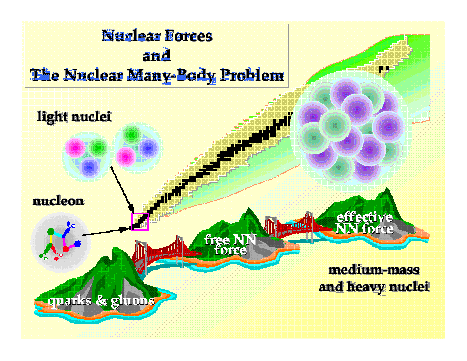A representation of the strong force. Depending on the complexity of the problem, scientists use different formulations.

The range of the gravitational and electromagnetic forces is infinite, while that of the strong and weak forces is very short. Also, the strength of the interaction depends on the separation between the two protons. Both gravitation and electromagnetism are of infinite range and their strengths decrease as the separation, r, increases–falling off as 1/r2. On the other hand, because the exchange particles for the strong and weak forces have a large mass, the force associated with them is zero outside of a short range.

Note that the strong force between two protons is a residual interaction. The quarks inside the nucleons interact through the exchange of gluons that carry a quantum number called "color" (i.e., a "color-neutral" object does not feel the strong force, like an electrically neutral object does not feel electromagnetism). Figure 4-1 shows three different ways that the strong force can be viewed.

The theory of strong interactions among quarks and gluons is called Quantum Chromodynamics (QCD). A very successful model of describing this residual force is by the exchange of the p (pi) meson, or pion. This description, dating back to the work of Yukawa in the 1930s, works extremely well except at very short distances, and is generally used to describe most of the properties of complex nuclei. Exactly at what distance inside the nucleus, or correspondingly at what values of momentum transferred between the nucleons, the pion exchange model breaks down is the subject of much current research in nuclear physics. It is presently thought that this transition occurs somewhere around momentum transfers of 1 GeV/c.

#### Chapter Contents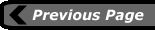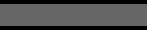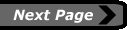last updated: August 9, 2000 webmaster